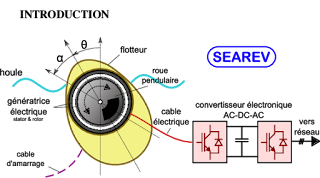## Articles

Affichage des articles du décembre, 2019

### Mine ventilation case calculationLet consider the following ventilation network in a mine. The network is made of five branches and four nodes.

The airflow which gets in the mine is 47 m3/s.

The Hardy Cross method will be used to compute the natural distribution of air flow in the different parts of the mine.

BranchRQ20.43030.61740.1350.52760.720
R is the airway resistance in kg/m7

1st case

We will start by considering an arbitrary distribution which respects the nodes law (the sum of incoming flows is equal to the sum of outgoing flows in a node)

If the distribution is real, the sum of head losses along any loop is zero.

The number of independent loops is given by b-n+1 where b is the number of branches and n the number of nodes.

In the present case the number of loops is 5-4+1=2

The chosen loops are 243 and  564. We walk along the loops clockwise. If the flow is in the same direction as the walk, the flow is considered positive. Otherwise it is negative.
LoopΣ R|Q|Q2Σ R|Q|Correction Δ2430.4 30^2 + 0.1 3^2 - 0.6 17^2…

### Répartition des débits d’air dans une mineSoit la mine suivante constituée de cinq branches et quatre noeuds

Le débit qui entre dans la mine est de 47 m3/s.

Nous allons utiliser la méthode de Hardy Cross pour trouver la répartition des débits dans les différents tronçons.

BrancheRQ20.43030.61740.1350.52760.720
R est la résistance aéraulique en kg/m7

1er cas

On commence par une distribution arbitraire qui respecte la loi des nœuds (somme des débits arrivant au nœud est égal à la somme sortant du nœud)

Si la répartition est la bonne, la somme des pertes de charges dans une boucle est nulle.

Le nombre de mailles m est donné par m=b-n+1 avec b nombre de branches et n nombre de nœuds.
Dans notre cas m=5-4+1=2

Les mailles choisies sont 243 et 564. On parcourt la maille dans le sens des aiguilles d’une montre, si le débit est dans le sens de parcours il est considéré positif sinon il est négatif.
MailleΣ R|Q|Q2Σ R|Q|Correction Δ2430.4 30^2 + 0.1 3^2 - 0.6 17^2=187.52(0.4 30 + 0.1 3 + 0.6 17)=45-4.1675640.5 27^2 - 0.7 20^2 - 0.1 3^2=83…

### TIPE 2020Le thème TIPE pour l'année 2019-2020 est intitulé Océan.

Dans ce qui suit quelques idées de sujets.

Les marées Etablissement du calendrier des marées à partir d'un modèle simple (modèle de l'océan global).

Ressources:

https://media4.obspm.fr/public/ressources_lu/pages_maree-terrestre/impression.html

https://vanoise49.pagesperso-orange.fr/annexe_5_forces%20de%20marees.html

Energie Houlomotrice Solutions pour extraire de l'énergie à partir des vagues. Dont voici quelques exemples:

Système SEAREV développé au début par l'école centrale de Nantes puis breveté par le CNRS

Le batteur de Salter ou « canard de Salter » par Stephen Salter. Le choc de la houle contre la partie effilée du batteur met en mouvement des roues à l'intérieur qui convertissent l'énergie en courant.

Système à colonne d'eau oscillante (Oscilating Water Column en anglais) Système de récupération de l'énergie à partir du mouvement des marées

Pelamis: structure semi-émergée composée de plusieurs …